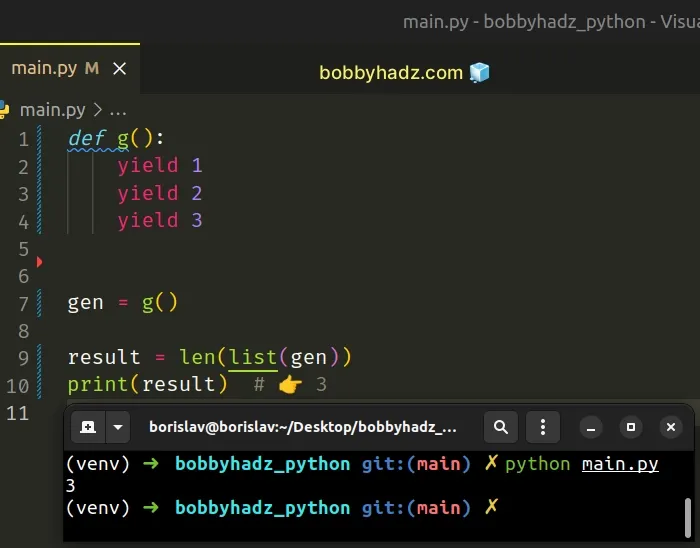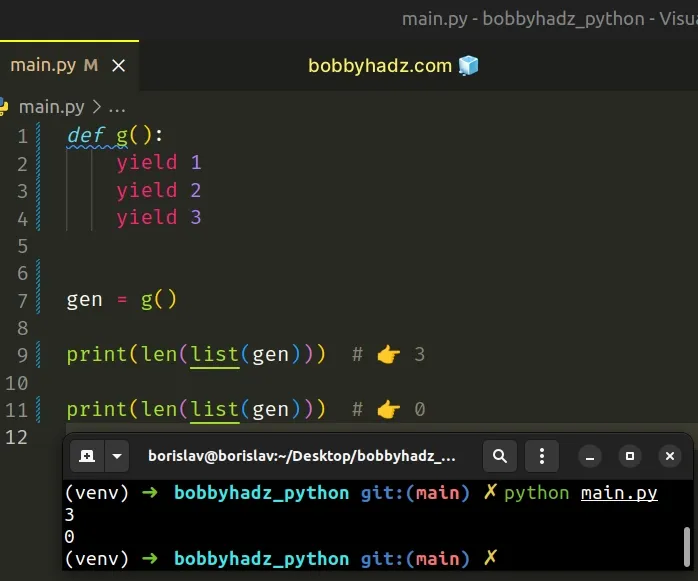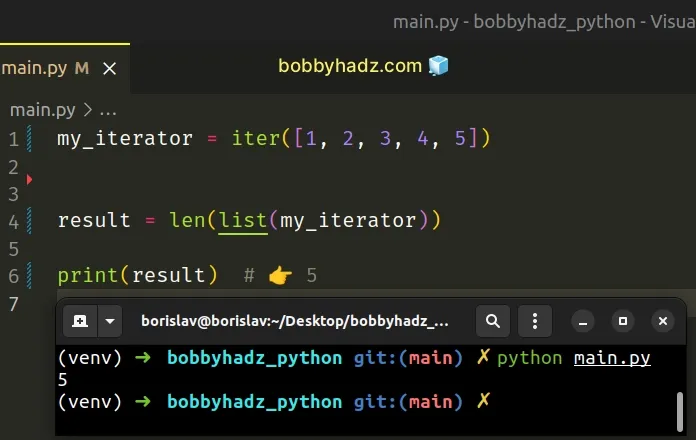# Get the length of a Generator or Iterator in PythonLast updated: Feb 18, 2023
3 min## #Get the length of a Generator in Python

To get the length of a generator in Python:

1. Use the `list()` class to convert the generator to a list.
2. Pass the list to the `len()` function, e.g. `len(list(gen))`.
3. Note that once the generator is converted to a list, it is exhausted.
main.py
```Copied!```def g():
yield 1
yield 2
yield 3

gen = g()

result = len(list(gen))
print(result)  # 👉️ 3
``````Note that once the generator is converted to a list it is exhausted.

main.py
```Copied!```def g():
yield 1
yield 2
yield 3

gen = g()

print(len(list(gen))) # 👉️ 3

print(len(list(gen)))  # 👉️ 0
``````You can convert the generator to a list and store the result in a variable.

main.py
```Copied!```def g():
yield 1
yield 2
yield 3

gen = g()

my_list = list(gen)

print(len(my_list))  # 👉️ 3
``````

Now you can iterate over the list as many times as necessary.

An alternative approach is to use the built-in `sum()` function.

main.py
```Copied!```def g():
yield 1
yield 2
yield 3

gen = g()

result = sum(1 for _ in gen)
print(result)  # 👉️ 3

print(list(gen))  # 👉️ []
``````

The sum function takes an iterable, sums its items from left to right and returns the total.

We effectively replace each item in the generator with `1` and sum the values.

This solution doesn't require us to convert the generator to a list and might be less memory-consuming.

However, the difference in performance is negligible unless you're dealing with generators with many millions of items.

This approach also exhausts the generator as there is no way to get the length of a generator without iterating over it.

Make sure you aren't trying to get the length of an infinite generator, as that operation would never complete.

## #Get the length of an Iterator in Python

The same approach can be used to get the length of an iterator.

main.py
```Copied!```my_iterator = iter([1, 2, 3, 4, 5])

result = len(list(my_iterator))

print(result)  # 👉️ 5
``````Note that once the iterator is converted to a list it is exhausted.

main.py
```Copied!```my_iterator = iter([1, 2, 3, 4, 5])

print(len(list(my_iterator)))  # 👉️ 5
print(len(list(my_iterator)))  # 👉️ 0
``````

You can convert the iterator to a list and store the result in a variable.

main.py
```Copied!```my_iterator = iter([1, 2, 3, 4, 5])

my_list = list(my_iterator)

print(len(my_list))  # 👉️ 5
``````

Now you can iterate over the list as many times as necessary.

An alternative approach is to use the built-in `sum()` function.

main.py
```Copied!```my_iterator = iter([1, 2, 3, 4, 5])

result = sum(1 for _ in my_iterator)

print(result)  # 👉️ 5
``````

The sum function takes an iterable, sums its elements from left to right and returns the total.

We effectively replace each item in the iterator with `1` and sum the values.

This solution doesn't require us to convert the iterator to a list and might be less memory-consuming.

However, the difference in performance is negligible unless you're dealing with iterators with many millions of elements.

This approach also exhausts the iterator as there is no way to get the length of an iterator without iterating over it.

Make sure you aren't trying to get the length of an infinite iterator, as that operation would never complete.